#Function Repository Resource:

# MultisetSum

Perform the sum operation on multisets

Contributed by: Robert B. Nachbar (Wolfram Solutions)
 ResourceFunction["MultisetSum"][list1,list2,…] gives a sorted list of all the elements that appear in any of the listi. The number of occurrences of each distinct element is the total of the counts in each of the listi. ResourceFunction["MultisetSum"][list] effectively sorts the elements of list. ResourceFunction["MultisetSum"][assoc1,assoc2,…] gives an Association where the keys are the union of the keys in the associations associ and the values are the sum of the merged values.

## Details and Options

If the listi are considered as multisets, ResourceFunction["MultisetSum"] gives their sum.
The listi must have the same head, but it need not be List.
The values of associ must be counts, that is, non-negative Integer values.

## Examples

### Basic Examples

Give a sorted list of elements from all the lists:

 In:=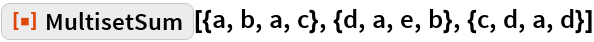Out=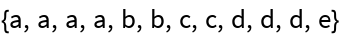Multisets as an Association of counts :

 In:=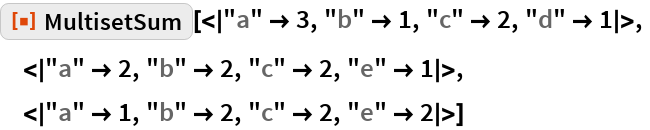Out=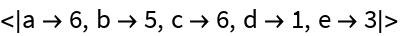### Scope

MultisetSum works with any head, not just List:

 In:=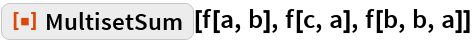Out=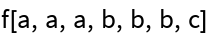### Properties and Relations

Compare to Union:

 In:=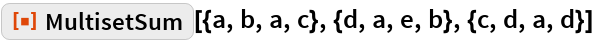Out=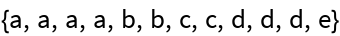In:=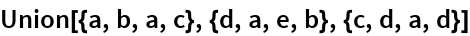Out=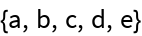## Requirements

Wolfram Language 11.3 (March 2018) or above